Home | About | Contact | Disclaimer | Help

Algebra

Equations

Inequalities

Graphs

Numbers

Calculus

Matrices

Tutorials

Enter the equation you want to plot, in terms of the variables x and y, set the limits and click the Plot button.

 Plot: from x = to x = from y = to y =

# Chapter 4 - QUADRATICS

## INTRODUCTION TO QUADRATICS

### Objectives

In this section, you will add, subtract, multiply, and graph quadratics.

Vocabulary: The standard format of a quadratic equation is y = ax2 + bx + c; a, b, c are constants; x is the independent variable, and y is the dependent variable. Quadratics are also called second degree polynomials because the highest exponent is 2. The slope-intercept equation from the second chapter, y = mx + b is called a first degree polynomial because the highest exponent is one.

Why study quadratics? The graphs of quadratic equations result in parabolas (U shaped graphs that open up or down). This feature of quadratics makes them good models for describing the path of an object in the air or describing the profit of a company (examples of which you may see in Finite Mathematics or in Microeconomics.)

Example 1. A boy lying on his back uses a sling shot to fire a rock straight up in the air with an initial velocity (the force the boy uses to fire the rock) of 64 feet per second. The quadratic equation that models the height of the rock is

h = -16t2 +64t.

a. Find the height of the rock when t = 0.

In the formula, h = -16t2 + 64t, replace t with 0.

h = -16(0)2 +64(0)
h = 0

The rock is zero feet in the air at zero seconds. (This is the point right before he shoots the rock in the air.)

b. Find the height of the rock when t = 1.

In the formula, h = -16t2 + 64t, replace t with 1.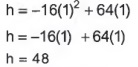The rock is 48 feet in the air at one second.

Explanation: Only the "1" is being squared. the -16 is multiplied by 12

c. Find the height of the rock when t = 2.

In the formula, h = -16t2 + 64t, replace t with 2.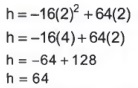The rock is 64 feet in the air at 2 seconds.

Explanation: Order of operations requires that you apply exponents before multiplying.

d. Find the height of the rock when t = 3.

In the formula, h = -16t2 + 64t, replace t with 3.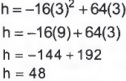The rock is 48 feet in the air at 3 seconds.

e. Find the height of the rock when t = 4.

In the formula, h = -16t2 +64t, replace t with 4.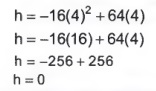The rock is zero feet in the air at 4 seconds; that is, the rock has hit the ground.

f. Graph the points obtained in parts a through e.

The height of the rock depends on the time, so h is the dependent variable, and t is the independent variable. The points have the form (t, h).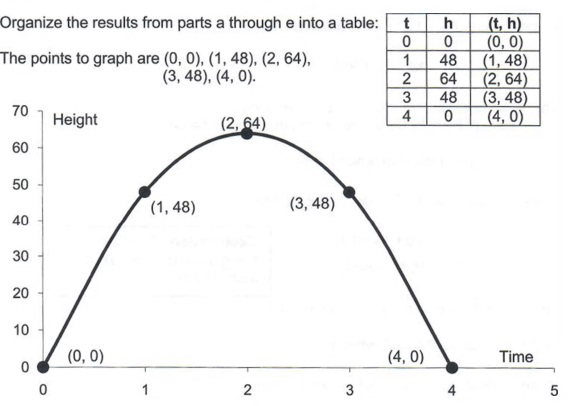According to the graph, the rock reaches its greatest height at 2 seconds. The maximum height is 64 feet. The maximum or minimum point of a quadratic is called the vertex. You will learn how to find the vertex in Section 4.3, Quadratic Applications and Graphs.

According to the graph, the rock is on the ground at zero seconds (right before the boy shoots it) and at 4 seconds (when the rock lands). These points are the time intercepts. You will learn how to find them in the next Section 4.2, "Applications of the Quadratic Formula."

Vocabulary: To add or subtract quadratics, combine like terms. Like terms, originally presented in the Section 1.3, "Simplifying Algebraic Expressions," have the same variable and the same exponent. For example 2x2 and 5x2 are like terms while 3x2 and 7x are not.

A coefficient, originally presented in Section 1.3, "Simplifying Algebraic Expressions," is the number multiplying the variable. For example, the coefficient of 2x is 2, and the coefficient of -x2 is -1.

Rule: To combine like terms, add their coefficients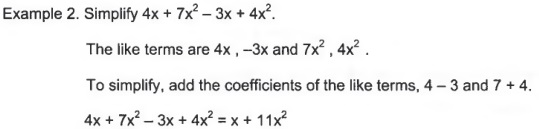Recall the distributive property: Definition a(b + c) = ab + ac.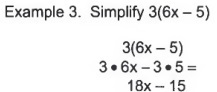Couldn't combine the unlike terms inside the parantheses so we used the distributive property. After that, we multiplied 6x by 3 and then -5 by 3.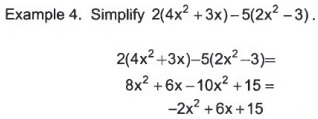Used the distributive property and combined like terms.

Example 5. The equation for profit is: Profit = Revenue - Cost

If the revenue equation for a company is: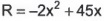and the cost equation is: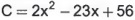find the profit equation for the company.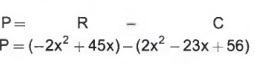Substituted the revenue and cost equations into the formula for profit. Must use parantheses.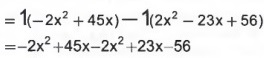Used the distributive property and multiplied the revenue equation by 1 and cost equation by -1.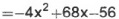Combined like terms.

Substituted the revenue and cost equations into the formula for profit. Must use parentheses. Used the distributive property. Multiplied the revenue equation by 1 and the cost equation by -1. Combined like terms.

Multiplying Two Binomials.

Vocabulary: A binomial has two terms (just as a bicycle has two wheels).

Rule: To multiply two binomials, multiply each term of the first by each term of the second.

Example 7. Multiply (x + 2)(5x + 3).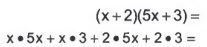Multiplied x by 5x and 3 and multiplied 2 by 5x and 3.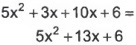Combine like terms.

FOIL is a simple mnemonic to remember how to multiply two binomials.

Example 8. Multiply (8x + 6)(x + 7).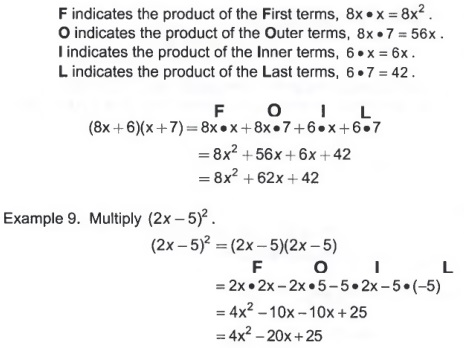Study Tip: Write a note card explaining the mnemonic FOIL. Review the card frequently.

### Summary

Quadratics are important equations in physics and microeconomics. The technique for adding and subtracting quadratics is the same as we have been practicing all semester; that is, add or subtract the like terms. To multiply, use the distributive property or FOIL. The vertex of the quadratic will be explained in more detail in the section, "Graphing Quadratics and Applications." The vertex is the maximum or minimum point on the graph of the quadratic.

## APPLICATIONS OF THE QUADRATIC FORMULA

### Objective

This section will show you how to solve quadratic equations.

Vocabulary: The quadratic equation is ax2 + bx + c = 0 . a, b and c are constants, and x is the variable.

The quadratic formula,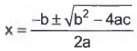, is used to solve a quadratic equation.

Analyzing• a, b, and c are numbers that will be substituted into the formula.
a is the coefficient of the squared variable,
b is the coefficient of the variable to the first power,
c is the constant.
• The symbol ± gives two solutions to the equation.
One solution is with the + sign,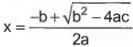and the other solution is with the - sign,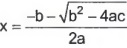.
•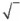is the square root symbol.
1. The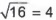because 42 = 16.
2. Thebecause 5.9162 = 35.
3. Your calculator is essential for this section. Make sure you can find the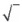button on your calculator.
4. You can only compute the square root of nonnegative numbers.
5. If you try to compute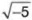your calculator will give an error message.
If you take Intermediate Algebra, you will learn about square roots of negative numbers.

Study Tip: Write the quadratic equation and quadratic formula on note cards, so you can reference them when you do your homework.

Example 1. Suppose you are standing on top of a cliff 375 feet above the canyon floor, and you throw a rock up in the air with an initial velocity of 82 feet per second. The equation that models the height of the rock above the canyon floor is:

h = -16t2 + 82t + 375.

Find how long it takes the rock to hit the canyon floor.

Find t when h = 0.
Solve 0 = -16t2 +82t + 375.
Indentify the constants a, b, and c.

Explanation: One side of the quadratic equation must be zero.

a = -16, b = 82, c = 375

Explanation:
a is the coefficient of the variable that is squared
b is the coefficient of the variable to the first power.
c is the constant.

Use the quadratic formula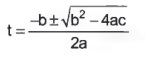with a = -16, b = 82, and c = 375.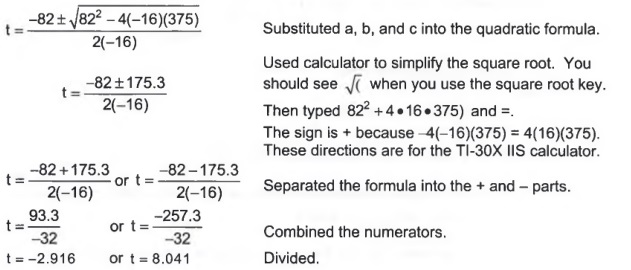T = -2.916 is a meaningless answer since t is the time it takes the rock to hit the canyon floor, and time cannot be negative.

T = 8.041 seconds is how long it takes the rock to hit canyon floor.

The rock will hit the canyon floor in 8.041 seconds.

Example 2. A rancher has 500 yards of fencing to enclose two adjacent pig pens that rest against the barn. If the area of the two pens must total 20,700 square yards, what should the dimensions of the pens be?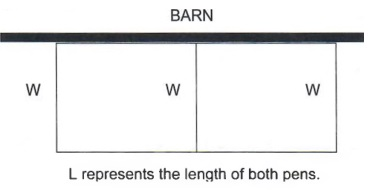L represents the length of both pens.

a. Use the table to find the equation for the area of the pens.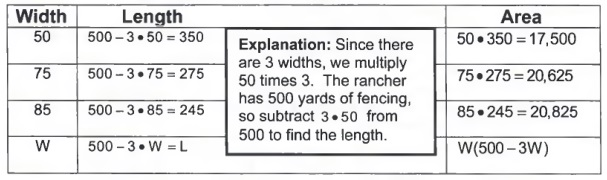b. Simplify the equation for area.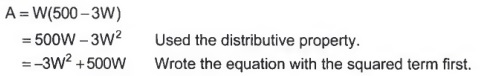c. Find W when A = 20,700.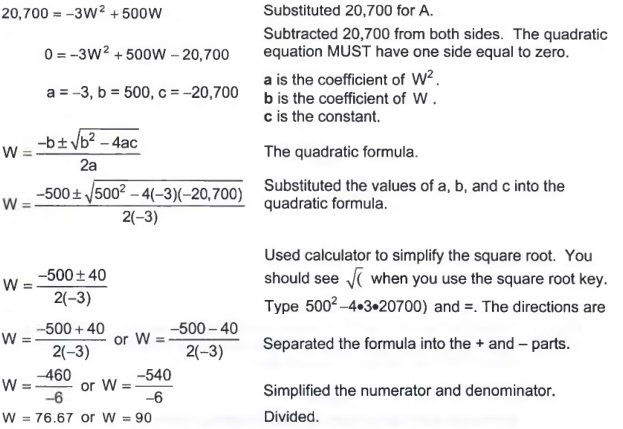The width is 76.67 or 90 yards.

d. Find the length of the pens.

From the table in Part a, L = 500 - 3W . Substitute W = 76.67 and W = 90 into the equation for length, L = 500 - 3w.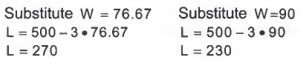The dimensions of the pig pens that yield an area of 20,700 square yards are 76.67 by 270 yards and 90 by 230 yards.

Example 3. During the course of an experiment, the temperature of oxygen must be monitored. Using the data from the experiment, the following quadratic can model the temperature of the oxygen,

T = 0.26m2 -4.1m + 7.9

where T is measured in Celsius, and m represents the minutes that the experiment has run. Determine when the temperature of the oxygen is 0 degree Celsius.

The problem asks you to find m when T = 0.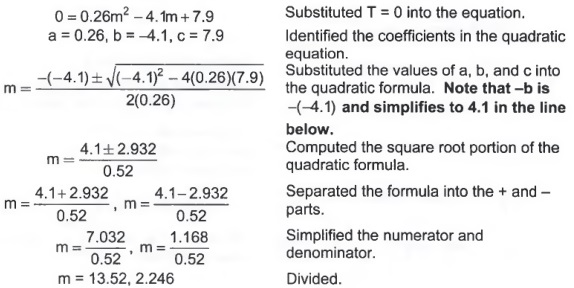The temperature of the oxygen will be 0 degrees Celsius in 2.246 minutes and 13.52 minutes.

Study Tip: The key idea demonstrated in example 3 is how to handle a negative b in the quadratic equation.

### Summary

This section shows us how to solve a new type of equation, the quadratic. These have important applications in many fields, such as business, physics, and engineering. Learn the difference between the quadratic equation and the quadratic formula.

The quadratic equation is ax2 + bx + c = 0.

• One side of the equation must be zero.
• a is the coefficient of x .
• b is the coefficient of x.
• c is the constant term.

The quadratic formula,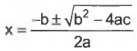solves the quadratic equation.

• The formula yields two solutions.
• The calculator is used to find the answers.
• The first step in evaluating the formula is to simplify the square root.

## QUADRATIC APPLICATIONS AND GRAPHS

### Objectives

This section explores further key points in the graph of a quadratic, the vertex and the intercepts. These points will be interpreted in applications.

Example 1. A boy lying on his back uses a sling shot to fire a rock straight up in the air with an initial velocity (the force the boy uses to shoot the rock) of 64 feet per second. The quadratic equation that models the height of the rock is

h = -16t2 + 64t.

(This example comes from Section 4.1 "Introduction to Quadratics", pg 317.)

On Page 318, we generated the following values: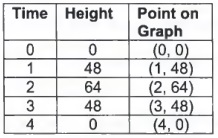We used the points to obtain the graph below. The vertex and intercepts are also labeled on the graph.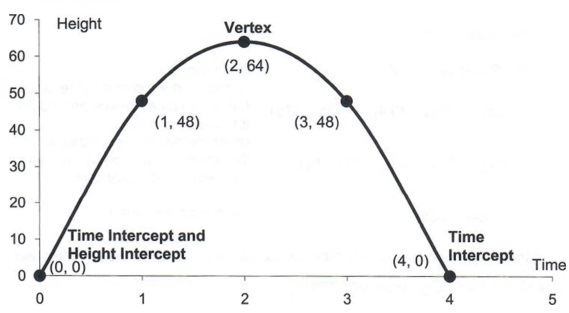Explanation: The point (0, 0) is both the time and height intercept.

The vertex, (2,64) represents the maximum height of the rock. The rock reaches a maximum height of 64 feet in 2 seconds.

The Time Intercepts, (0, 0) and (4, 0) represent when the rock is on the ground. The rock is on the ground at zero seconds, before it is shot, (which is the Height Intercept) and at 4 seconds, when it returns to the ground.

To graph a quadratic indicated by the equation, y = ax2 + bx + c, master the following terms:

Vocabulary: Vertex: The vertex is the maximum or minimum point on the graph. To find the vertex :

a. Find the x coordinate: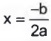b. Find the y coordinate: Substitute the value for x obtained in Part a into the formula y = ax2 + bx + c.

X intercept: Set y = 0 and solve 0 = ax2 + bx + c using the quadratic formula,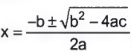Y intercept: Set x = 0 and find y. y will always be c, the constant.

Study Tip: Write the procedure and definitions on three note cards for easy reference.

Example 2. The company D+++ makes computer games. The cost of making g games per month is C = 0.4g2 - 32g + 625 . The revenue from selling g games per month is R = -0.6g2 +52g. The units for g are in hundreds, and C and R are in thousands of dollars.

a. Find the profit equation.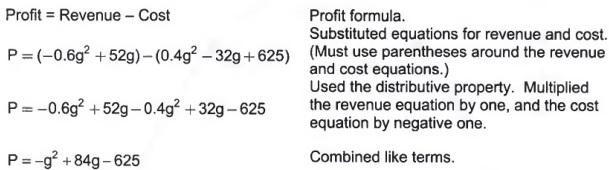b. Find the vertex and explain what the vertex means in terms of making computer games.

The formula for the g coordinate is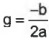From the equation for profit, a = -1, b = 84.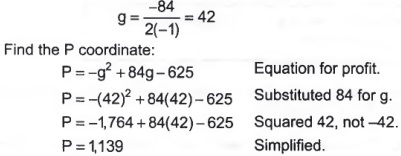The vertex is (42,1139). If D+++ sells 4,200 games, then they will earn a maximum profit of \$1,139,000.

c. Find the g intercepts and explain what they mean in terms of making computer games.

To find the g intercept, set P = 0.

Solve 0 = -g2 + 84g - 625 .

Use the quadratic formula, a = -1, b = 84, c = -625.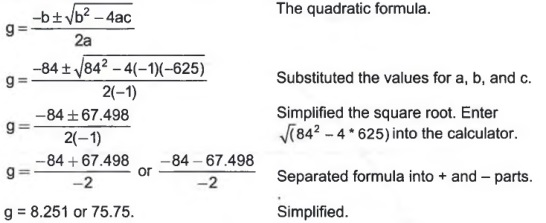The g intercepts are (8.251, 0) and (75.75, 0).

If they sell 825 or 7,575 games, they will break even.

d. Find the P intercepts and explain what they mean in terms of making computer games.

To find the P intercept, set g = 0.
P = -02 +84*0-625
P = -625
The P intercept is (0, -625).
The company's start up costs are \$625,000.

e. Graph the function.

Plot the points:
Vertex. (42, 1139).
The g intercepts. (8.251, 0) and (75.75, 0).
The P intercept. (0, -625).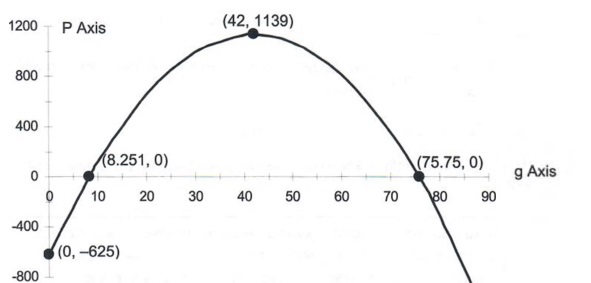Explanation: One explanation for the profit having two break even points is how efficient a company is at making a product. Making very few items is usually inefficient. At some point, the factory becomes very efficient at manufacturing the product, but if the factory tries to make too many items, the company becomes inefficient at producing its product.

Remember that the units for g are in hundreds, and the units for P are thousands.

Suppose D+++ needs to make a profit of \$500,000 (P = 500) a month. Sketch this line on the graph obtained in Part b and find where the line intersects the graph of the quadratic. Write a sentence explaining what the answers mean.

Sketch P = 500 on the previous graph.

P = 500 is a horizontal line.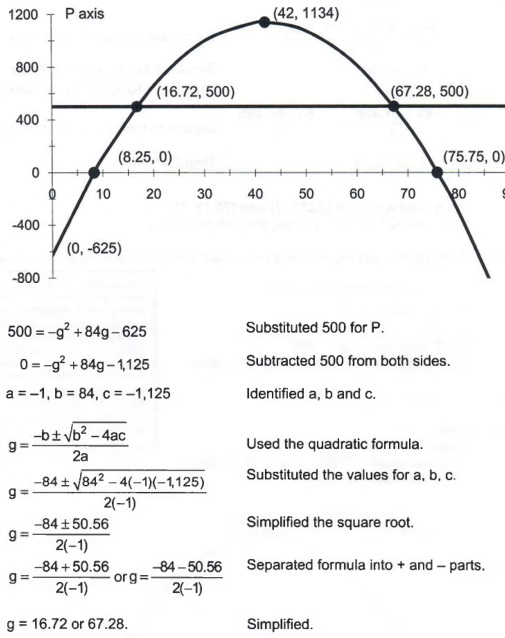If D+++ wants a profit of \$500,000, then they need to make and sell 1,672 or 6,728 games.

Explanation: The graph gives an estimate of where the horizontal line, P = 500, and the equation for profit, P = -g2 +84g-625 intersect. Algebra gives the exact point where they intersect.

g. Using the graph and the answers to Part c, determine how many computer games must be made and sold to guarantee a profit greater than \$500,000.

The company will earn a profit of more than \$500,000 when the profit graph is above the horizontal line P = 500. This problem is similar to example 2d on page 203 in Section 2.9 "Applications of Graphs".

This occurs between the points g = 16.72 and g = 67.28 or

16.72 < g < 67.28.

The company will earn more than \$500,000 when they make and sell between 1,672 and 6,728 computer games.

Example 3. A kennel operator wants to enclose three adjacent dog pens of equal size against a wall. He has 96 meters of fence.

a. Find the formula for area.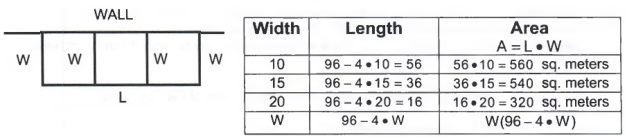Explanation: The most difficult part of the table is finding the value for length. If the farmer uses 10 meters for the width of the pens, and there are 4 widths, then he has used 4 times 10 , or 40 meters of fencing. To find how much fencing he has left for the length, subtract 40 from 96, the total amount of fencing available to the farmer.

The formula for the area of the dog pens is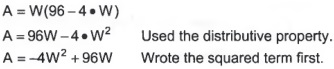b. Find the vertex and explain what it means in terms of dog pens.

The formula for the W coordinate is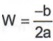From the equation for profit, a = -4, b = 96.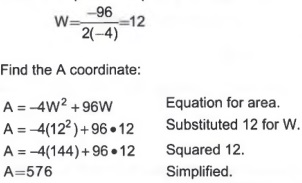The vertex is (12, 576).

The vertex, (12, 576) represents the maximum area of the three dog pens. When W = 12, the maximum area will be 576. (The length of all three pens will be 48 or the length of one dog pen will be 16.) There will be three dog pens each 12 by 16 meters.

c. Find the W intercepts and explain what they mean in term of the dog pens.

To find the W intercept, set A = 0.

Solve 0 = -4W2 + 96W.

Use the quadratic formula, a = -4, b = 96, c = 0.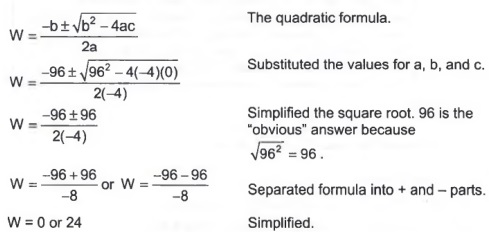The W intercepts are (0, 0) and (24, 0).

The W intercepts, (0, 0) and (24, 0) represent the widths of the dog pens that will yield zero area.

d. Find the A intercept and explain what it means in terms of the dog pens.

To find the A intercept, set W = 0.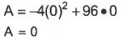Explanation: If the width of a rectangle is zero, then the area has to be zero.

The A intercept is (0, 0).

The A intercept, (0, 0) is the area when W = 0.

e. Graph the equation

Plot the points:
Vertex. (12, 576).
The W intercepts. (0, 0) and (24, 0).
The A intercept. (0, 0).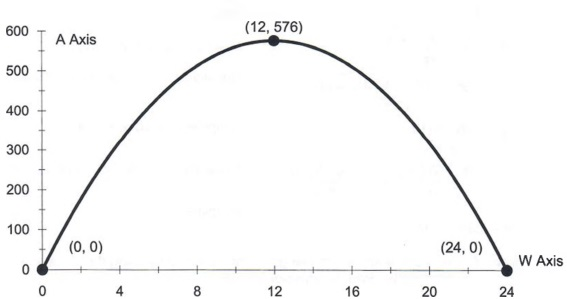f. Suppose the total area has to be 400 square meters. Graph A = 400 and find the dimensions of the dog pens.

Sketch A = 400 on the previous graph.

A = 400 is a horizontal line.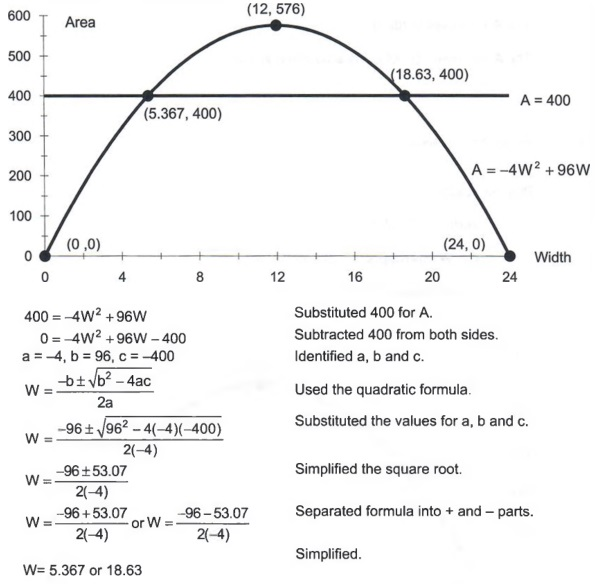Since W, the width, is known, the length L can be found by using the formula A = LW.

Solve for L by dividing both sides by W.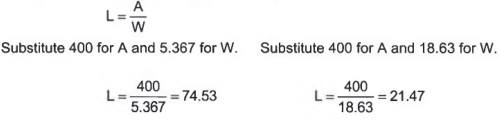The dimensions of the dog pens that will give an area of 400 square meters are 5.367 by 74.53 and 18.63 by 21.47.

Example 4. During the course of an experiment, the temperature of oxygen must be monitored. Using the data from the experiment, the following quadratic can model the temperature of the oxygen,

T = 0.26m2 -4.1m + 7.9

where T is measured in Celsius, and m represents the minutes that the experiment has run. Graph the equation by finding the vertex and the intercepts. Label these points on the graph and explain what the vertex and intercepts mean in terms of the model.

Go Back: This is the same model that was used in Example 3 on page 332. That example was worked when the temperature was zero.

Find the vertex of T = 0.26m2 - 4.1m + 7.9 .

The formula for the m coordinate of the vertex is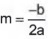.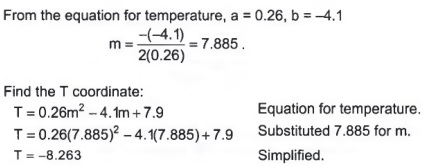The vertex is (7.885, -8.263).

Find the m intercepts of T = 0.26m2 -4.1m+ 7.9

To find the m intercepts, set T = 0.

Solve 0 = 0.26m2-4.1m+ 7.9 .

Use the quadratic formula, a = 0.26, b = -4.1, c = 7.9.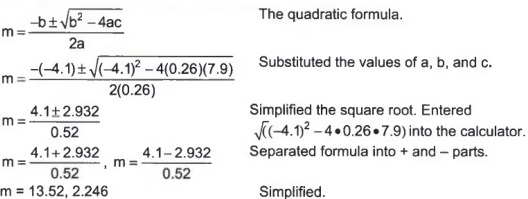The m intercepts are (13.52, 0) and (2.246, 0).

Find the T intercepts of T = 0.26m2 - 4.1m + 7.9

To find the T intercept, set m = 0.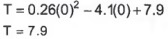The T intercept is (0, 7.9).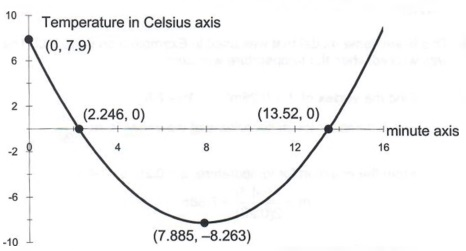Vertex: The temperature will be a minimum at 7.885 minutes. The minimum temperature will be -8.263 degrees Celsius.

m intercepts: The temperature will be zero degrees Celsius at 2.246 and 13.52 minutes.

T intercept: The temperature was 7.9 degrees Celsius at the start of the experiment.

Study Tips: Quadratics are U shaped graphs. In some cases, they are U shaped as in the example above or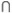shaped as in examples 1 through 3. If a in the equation, y = ax2 + bx + c, is positive, then the graph is U shaped, that is, opening up. If a is negative, the graph isshaped, that is, opening down. This fact should be written on a note card.

### Summary

Graphs of quadratics appear in subjects as diverse as microeconomics and physics. This section summarizes the major ideas of the unit.

To graph a quadratic, y = ax2 + bx + c , you should find:

• The vertex.
The formula for the x coordinate is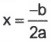To find the y coordinate, substitute your answer for the x coordinate in the equation y = ax2 + bx + c .
• The x intercepts. Set y = 0 and solve the equation, 0 = ax2 + bx + c , using the quadratic formula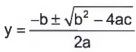• The y intercept.
Set x = 0 in the equation, y = ax2 + bx + c , and find y. Note, when x = 0, y = c.
• If a is negative, generally the graph looks like this: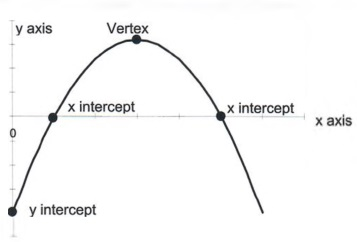• If a is positive, generally the graph looks like this: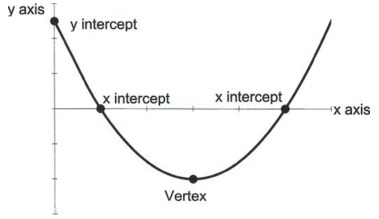## FACTORING

### Objectives

Factoring is an algebraic technique used to separate an expression into its component parts. When the component parts are multiplied together, the result is the original expression. This can sometimes be used to solve quadratic equations. Factoring is an important skill in MAT 100, Intermediate Algebra.

Vocabulary: An algebraic expression is factored if the last operation in evaluating the expression is multiplication.

Example 1. Which expression is factored, x2 - 5x - 24 or (x - 8)(x + 3)?

Pick a value for x and substitute it into the expression.

Let x = 3.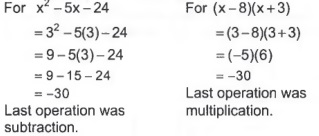Since the last operation for(x - 8)(x + 3) was multiplication, then (x - 8)(x + 3) is factored.

Explanation: Less formally, an algebraic expression is factored when it has parentheses.

Vocabulary: The distributive property is a(b + c) = ab + ac. The left hand side is factored and a is the common factor.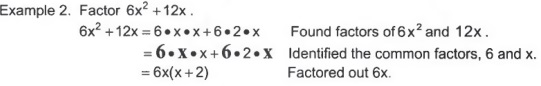You should be able to check by using the distributive property.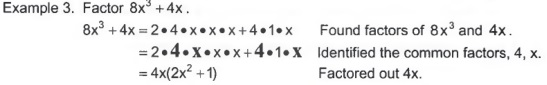Explanation: Although 8x3 + 4x is equal to both 2x(4x2 + 2) and 4(2x3 + x) neither are considered completely factored because in both cases a common multiple, 2, in 2x(4x2 +2) and x in 4(2x3 +x) can still be factored from the terms in the parenthesis.

Factoring Trinomials: (A trinomial has three terms.) To factor a trinomial, recall the acronym FOIL.

Study Tip: Check your note cards for the definition of FOIL.

Example 4. Multiply (x+3)(x+5).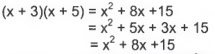(x+3)(x+5) is factored while x2 + 8x +15 is not. To factor trinomials, you need to know how the 8x and the 15 were computed. The 8x came from adding 5x and 3x while 15 came from multiplying 5 and 3.

Example 5. Factor x2 + 8x +15. (This is from Example 4.)

We need two numbers that when added equal 8 and when multiplied equal 15. 3 and 5 add up to 8 and when multiplied are 15.

So x2 + 8x +15 = (x + 3)(x + 5)

Example 6. Factor x2-4x- 12.

We need two numbers that when added equal -4 and when multiplied equal -12. -6 and 2 add up to -4 and when multiplied are -12.

So x2 -4x -12 = (x-6)(x + 2).

Example 7. Factor x2 - 64 .

This is not a trinomial, but it can become one by adding 0x.

x2 -64 = x2 +0x -64

We need two numbers that when added equal 0 and when multiplied equal -64.

-8 and 8 add to 0 and when multiplied are -64.

So x2 -64 = (x-8)(x + 8).

This example is called factoring the difference of perfect squares, and you will see this again if you take MAT 100, Intermediate Algebra.

Vocabulary: a2 - b2 is the difference of perfect squares.
The difference of perfect squares has a special factoring formula: a2 - b2 = (a - b)(a + b)

Solving Quadratic Equations by Factoring:

If you multiply two quantities and the result is zero, then you know that one of the quantities must be zero. In mathematical notation

if a.b = 0 then a = 0 or b = 0 .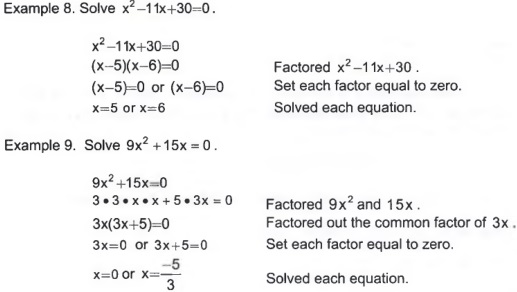Before you think that factoring to solve quadratics is a lot easier than using the quadratic formula, you need to know that factoring doesn't always work. Consider changing Example 8 by just one to x2 - 11x + 31 = 0. You cannot find two integers that when added equal -11 and when multiplied equal 31. To factor x2 - 11x + 31 you must use the quadratic formula. You will learn how to factor any quadratic equation in Precalculus I, MAT 161.

### Summary

Two techniques for factoring are presented in this unit. The first is common factors which uses the distributive property, ab + ac = a(b + c). The other one is factoring trinomials. To factor trinomials, you need to know how FOIL works. If you take MAT 100, Intermediate Algebra, you will see more factoring.

## CHAPTER 4 REVIEW

This chapter introduced you to quadratics. The two major topics are the quadratic formula and graphs of quadratics. These topics have many applications in business, physics, and geometry. Factoring is an important topic in MAT 100, Intermediate Algebra.

### Section 4.1: Introduction to Quadratics### Section 4.2: Applications of the Quadratic Formula

Definition: ax2 + bx + c = 0 is the quadratic equation.

Definition: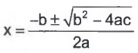is the quadratic formula.

Example 4. A farmer wants to enclose two adjacent chicken coops against a barn. He has 125 feet of fence. What should the dimensions be if he wants the total area to be 700 square feet.

a. Complete the table to find the equation for area.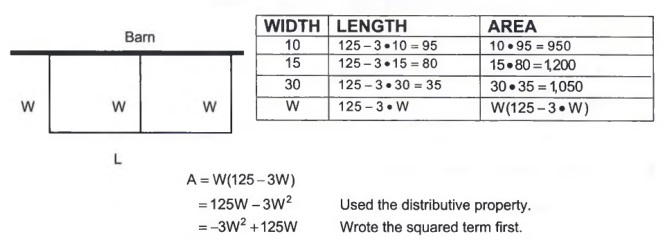b. Find W when A = 700.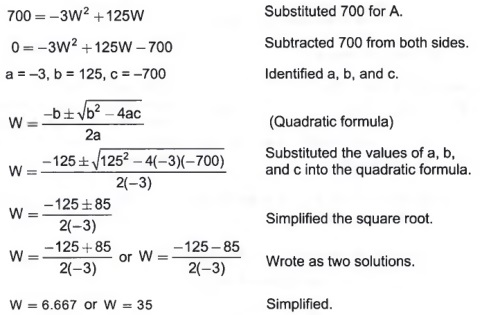The dimensions of the chicken coop that will yield an area of 700 square feet are 35 by 20 feet and 6.667 by 105 feet.
(To get the length divide 700 by 6.667 and 35.)

### Section 4.3: Quadratic Applications and Graphs

To graph a quadratic, y = ax2 + bx + c you must find:

1. The vertex:
The x coordinate is computed with the formula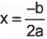The y coordinate is computed by substituting the x coordinate into y = ax2 + bx + c.
2. The x intercept:
Set y = 0 and solve 0 = ax2 + bx + c using the quadratic formula.
3. The y intercept:
Substitute x = 0 into y = ax2 + bx + c . Note that when x = 0, y = c.

Example 5. The cost equation for making juice boxes is C = 0.6B2 - 24B + 36, and the revenue equation is R = -0.4B2 + 18B . B is in millions, and C and R are in thousands of dollars.

a. Find the profit equation.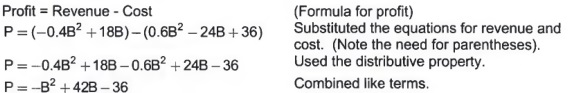b. Graph the profit equation and explain what the vertex, B, and P intercepts mean in terms of the problem.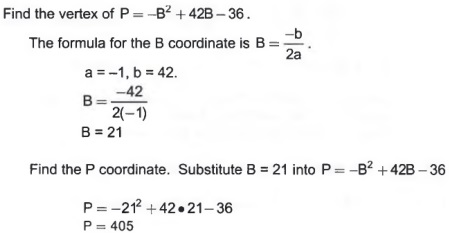The vertex is (21, 405).

Find the B intercept. Set P = 0.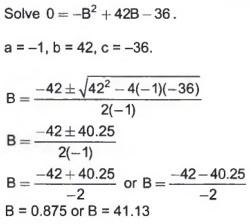The B intercepts are (0.875, 0) and (41.13, 0).

Find the P intercept. Set B = 0.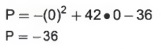The P intercept is (0, -36).

c. Suppose the company needs to earn \$200,000 in profit (P = 200). Graph the line P = 200 and find how many juice boxes the company needs to make to earn \$200,000.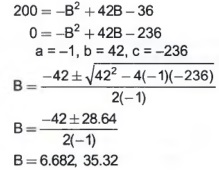The company needs to make 6.682 or 35.32 million juice boxes in order to earn \$200,000 in profits.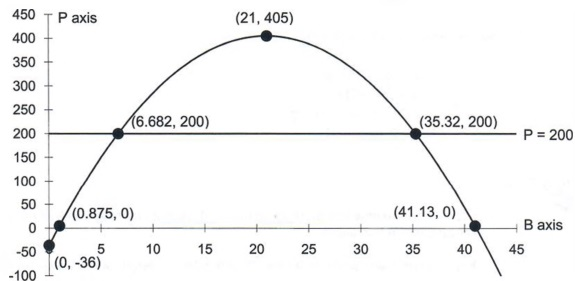The vertex (21,405) represents the maximum profit. The company will obtain its maximum profit of \$405,000 when they sell 21 million juice boxes.

The B intercepts (0.875, 0) and (41.13, 0) tell us that the company will break even if they sell .875 or 41.13 million juice boxes.

The P intercept (0. -36) represents the company's start up costs of \$36,000.

### Section 4.4: Factoring

Common Factors: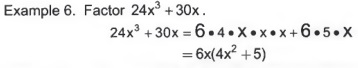Trinomials: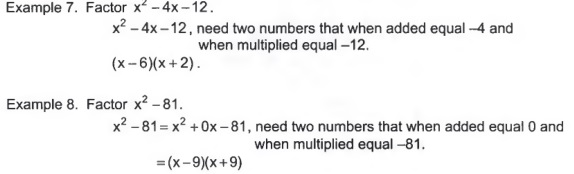Solving quadratic equations by factoring.

If a . b = 0 then a = 0 or b = 0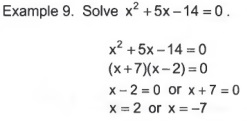Study Tips:

1. Practice the review test starting on the next page by placing yourself under realistic exam conditions.
2. Find a quiet place and use a timer to simulate the length of the class period.
3. Write your answers in your homework notebook or make a copy of the test. You may then re-take the exam for extra practice.